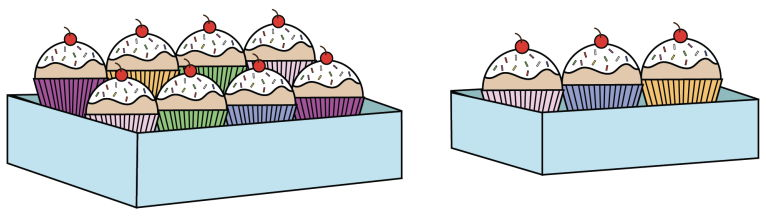시간 제한메모리 제한제출정답맞힌 사람정답 비율
1 초 1024 MB18431264120268.295%

## 문제

A regular box of cupcakes holds 8 cupcakes, while a small box holds 3 cupcakes. There are 28 students in a class and a total of at least 28 cupcakes. Your job is to determine how many cupcakes will be left over if each student gets one cupcake.## 입력

The input consists of two lines.

• The first line contains an integer R ≥ 0, representing the number of regular boxes.
• The second line contains an integer S ≥ 0, representing the number of small boxes.

## 출력

Output the number of cupcakes that are left over.

## 예제 입력 1

2
5


## 예제 출력 1

3


The total number of cupcakes is 2 × 8 + 5 × 3 which equals 31. Since there are 28 students, there are 3 cupcakes left over.

## 예제 입력 2

2
4


## 예제 출력 2

0


The total number of cupcakes is 2 × 8 + 4 × 3 which equals 28. Since there are 28 students, there are no cupcakes left over.